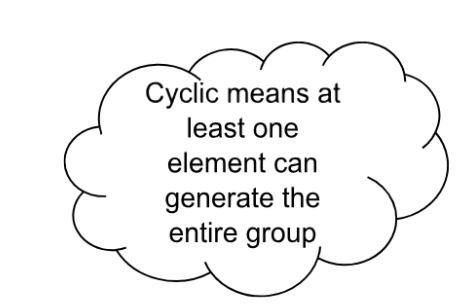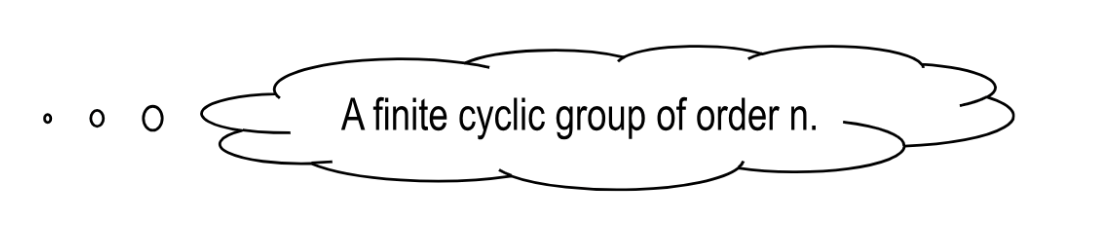# 2.4: Introduction to cyclic groups

$$\newcommand{\vecs}{\overset { \rightharpoonup} {\mathbf{#1}} }$$ $$\newcommand{\vecd}{\overset{-\!-\!\rightharpoonup}{\vphantom{a}\smash {#1}}}$$$$\newcommand{\id}{\mathrm{id}}$$ $$\newcommand{\Span}{\mathrm{span}}$$ $$\newcommand{\kernel}{\mathrm{null}\,}$$ $$\newcommand{\range}{\mathrm{range}\,}$$ $$\newcommand{\RealPart}{\mathrm{Re}}$$ $$\newcommand{\ImaginaryPart}{\mathrm{Im}}$$ $$\newcommand{\Argument}{\mathrm{Arg}}$$ $$\newcommand{\norm}{\| #1 \|}$$ $$\newcommand{\inner}{\langle #1, #2 \rangle}$$ $$\newcommand{\Span}{\mathrm{span}}$$ $$\newcommand{\id}{\mathrm{id}}$$ $$\newcommand{\Span}{\mathrm{span}}$$ $$\newcommand{\kernel}{\mathrm{null}\,}$$ $$\newcommand{\range}{\mathrm{range}\,}$$ $$\newcommand{\RealPart}{\mathrm{Re}}$$ $$\newcommand{\ImaginaryPart}{\mathrm{Im}}$$ $$\newcommand{\Argument}{\mathrm{Arg}}$$ $$\newcommand{\norm}{\| #1 \|}$$ $$\newcommand{\inner}{\langle #1, #2 \rangle}$$ $$\newcommand{\Span}{\mathrm{span}}$$$$\newcommand{\AA}{\unicode[.8,0]{x212B}}$$

##### Definition: order of an element

Let $$(G,\star)$$ be a group.

Let $$a \in G$$, then the order of the element $$a \in G$$, be the smallest positive integer $$n$$ s.t. $$a^n=e$$.  If no such $$n$$ exists, we say that the order is infinite.

##### Definition:

Let $$(G,\star)$$ be a group.

Let $$a \in G$$, then define $$<a>=\{a^m|m\in \mathbb{Z}\}$$.

##### Theorem $$\PageIndex{1}$$

Let $$(G,\star)$$ be a group.

Let $$a \in G$$, then

$$<a> \leq G.$$

##### Example $$\PageIndex{1}$$

Consider the group $$\mathbb{Z}_{10}$$ with addition modulo 10.  What is the order of its elements?

Consider that $$\mathbb{Z}_{10}=\{0,1,2,3,4,5,6,7,8,9\}$$.   Note that $$\{0\}$$ is the identity and its order is $$1.$$

 element Order Calculations 0 $$|<0>|=1$$ 1 $$|<1>|=10$$ $$1^{10}\equiv 0 \pmod{10}$$ 2 $$|<2>|=5$$ $$2^{1}\equiv 2\pmod{10}$$, $$2^2 \equiv 4 \pmod{10}$$, $$2^{3}\equiv 6 \pmod{10}$$, $$2^{4} \equiv 8 \pmod{10}$$, and $$2^{5}\equiv 0 \pmod{10}$$. 3 $$|<3>|=10$$ $$3^1\equiv 3 \pmod{10}$$, $$3^2\equiv 6 \pmod{10}$$ , $$3^3\equiv 9 \pmod{10}$$, $$3^4\equiv 2 \pmod{10}$$, $$3^5\equiv 5 \pmod{10}$$, $$3^6\equiv 8 \pmod{10}$$, $$3^7\equiv 1 \pmod{10}$$, $$3^8\equiv 4 \pmod{10}$$, $$3^9\equiv 7 \pmod{10}$$, and  $$3^{10}\equiv 0 \pmod{10}$$. 4 $$|<4>|=5$$ $$4^1 \equiv 4 \pmod{10}$$, $$4^2 \equiv 8 \pmod{10}$$, $$4^3 \equiv 2 \pmod{10}$$, $$4^4 \equiv 6 \pmod{10}$$, and $$4^5 \equiv 0 \pmod{10}$$. 5 $$|<5>|=2$$ $$5^1 \equiv 5 \pmod{10}$$, and $$5^2 \equiv 0 \pmod{10}$$. 6 $$|<6>|=5$$ $$6^1 \equiv 6 \pmod{10}$$, $$6^2 \equiv 2 \pmod{10}$$, $$6^3 \equiv 8 \pmod{10}$$, $$6^4 \equiv 4 \pmod{10}$$ and $$6^5 \equiv 0 \pmod{10}$$. 7 $$|<7>|=10$$ $$7^1 \equiv 7 \pmod{10}$$, $$7^2 \equiv 4 \pmod{10}$$, $$7^3 \equiv 1 \pmod{10}$$, $$7^4 \equiv 8 \pmod{10}$$, $$7^5 \equiv 5 \pmod{10}$$, $$7^6 \equiv 2 \pmod{10}$$, $$7^7 \equiv 9 \pmod{10}$$, $$7^8 \equiv 6 \pmod{10}$$, $$7^9 \equiv 3 \pmod{10}$$, and $$7^{10} \equiv 0 \pmod{10}$$. 8 $$|<8>|=5$$ $$8^1 \equiv 8 \pmod{10}$$, $$8^2 \equiv 6 \pmod{10}$$, $$8^3 \equiv 4 \pmod{10}$$, $$8^4 \equiv 2 \pmod{10}$$, and  $$8^5 \equiv 0 \pmod{10}$$. 9 $$|<9>|=10$$ $$9^1 \equiv 9 \pmod{10}$$, $$9^2 \equiv 8 \pmod{10}$$, $$9^3 \equiv 7 \pmod{10}$$, $$9^4 \equiv 6 \pmod{10}$$, $$9^5 \equiv 5 \pmod{10}$$, $$9^6 \equiv 4 \pmod{10}$$, $$9^7 \equiv 3 \pmod{10}$$, $$9^8 \equiv 2 \pmod{10}$$, $$9^9 \equiv 1 \pmod{10}$$,  and $$9^10 \equiv 0 \pmod{10}$$.

##### Note

Let $$(G,\star)$$ be a group.Let $$a \in G$$, then $$<a>$$ is called cyclic subgroup of $$G.$$

##### Definition: Cyclic subgroup

If  $$G=<a>$$  for some $$a \in G$$, then $$G$$ is called a cyclic group.

##### Example $$\PageIndex{1}$$

$$U(15)=\{1,2,4,7,8,11,13,14\}$$ and $$\star= \bullet \pmod{15}$$.

Let $$a \in U(15)$$ and $$b \in U(15)$$.

Then $$a(x)+15(y)=1$$ and $$ab(x)+15by=b$$  therefore $$U(15)$$ is closed.Since $$U(15)$$ is a finite group, the order of the group is $$|U(15)|=8$$.

However, $$U(15)$$ is not a cyclic group.

Proof: (by exhaustion)

Let $$a \in U(15)$$.

We will show that $$\not \exists a \in U(15)$$, s.t. $$|<a>|=8$$.

$$|<1>|=1$$ since $$1^1 \equiv 1 \pmod{15}$$

$$|<2>|=4$$ since $$2^4 \equiv 1 \pmod{15}$$

$$|<4>|=2$$ since $$4^2 \equiv 1 \pmod{15}$$

$$|<7>|=4$$ since $$7^4 \equiv 1 \pmod{15}$$.

$$|<8>|=4$$ since $$8^4 \equiv 1 \pmod{15}$$.

$$|<11>|=2$$ since $$11^2 \equiv 1 \pmod{15}$$.

$$|<13>|=4$$ since $$13^4 \equiv 1 \pmod{15}$$.

$$|<14>|=2$$ since $$14^2 \equiv 1 \pmod{15}$$.

Since $$\not \exists a \in U(15)$$, s.t. $$|<a>|=8$$, $$U(15)$$ is not a cyclic group.◻

##### Example $$\PageIndex{2}$$

Prove that $$(U(14),(\bullet \pmod{14}))$$ is cyclic.Proof:

We will show that $$\exists a \in U(14)$$ s.t. $$|<a>|=|U(14)|$$.

Consider  $$U(14)=\{1,3,5,9,11,13\}$$ where $$|U(14)|=6$$.

Consider $$3^1\equiv 3 \pmod{14}$$, $$3^2\equiv 9 \pmod{14}$$, $$3^3 \equiv 13 \pmod{14}$$,  $$3^4\equiv 11 \pmod{14}$$,  $$3^5\equiv 5 \pmod{14}$$ and $$3^6 \equiv 1 \pmod{14}$$.

$$|<3>|=6$$ since $$3^6 \equiv 1 \pmod{14}$$.

Thus $$|<3>|=6$$.

Therefore, $$U(14)$$ is cyclic and $$3$$ is a generator.◻

##### Example $$\PageIndex{1}$$

Is $$(\mathbb{Z},+)$$ cyclic group?  If so, what are the possible generators?

Yes, $$(\mathbb{Z},+)$$ is a cyclic group that is generated by $$\pm 1$$.

Proof of being a cyclic group:

Since the group generated by $$1$$ contains $$1,$$  the identity  $$0,$$  and the inverse of $$1, (-1),$$ as well as all multiples of $$1$$ and $$(-1)$$, $$(\mathbb{Z},+)$$ is cyclic.

Possible Generators:

Note $$1^n$$ is $$1+1+\cdots+1$$ with $$n$$ terms when $$n>0$$ and $$(-1)+(-1)+\cdots+(-1)$$ with $$n$$ terms when $$n$$ is $$<0$$.

We shall show $$\mathbb{Z}=<1>=<-1>$$.

Consider that $$1^n$$ means $$1+1+\cdots +1$$ with $$n$$ terms and that $$1^{-n}$$ means $$+(-1)+(-1)+\cdots+(-1)$$ with $$n$$ terms.

It should be clear that $$1^n$$ will generate $$\mathbb{Z}_+$$ and that $$1^{-n}$$ will generate $$\mathbb{Z}_-$$.  Note that $$1^0$$ is interpreted as $$0\cdot 1=0$$.

$$<1>=\{ \ldots, -3,-2,-1,0,1,2,3,\ldots \}$$.

Thus $$<1>$$ is a generator of $$(\mathbb{Z},+)$$.

Similarly, we will show that $$<-1>$$ is a generator of $$(\mathbb{Z},+)$$.

Consider that $$(-1)^n$$ means $$+(-1)+(-1)+\cdots+(-1)$$ with $$n$$ terms and that $$1^{-n}$$ means $$1+1+\cdots +1$$ with $$n$$ terms.

It should be clear that $$1^n$$ will generate $$\mathbb{Z}_-$$ and that $$1^{-n}$$ will generate $$\mathbb{Z}_+$$.  $$<-1>=\{ \ldots, -3,-2,-1,0,1,2,3,\ldots \}$$.

Thus the generators of $$(\mathbb{Z},+)$$ are $$<1>$$ and $$<-1>$$.

## Properties:

##### Theorem $$\PageIndex{2}$$

Cyclic groups are abelian.

Proof:

Let $$(G,\star)$$ be a group.

If $$G$$ is cyclic, then $$G$$ is abelian.

Assume that $$G$$ is cyclic.

Then $$G=<g>$$ for some $$g \in G$$.

Let $$a,b \in G$$.

We will show $$ab=ba$$.

Then $$a=g^m$$ and $$b=g^n$$, $$m,n \in \mathbb{Z}$$.

Consider $$ab=g^mg^n$$

$$=g^{m+n}$$

$$=g^{n+m}$$

$$=g^ng^m$$

$$=ba$$

Hence $$G$$ is abelian.◻

##### Note

The converse is not true.  Specifically, if a group is abelian, it is not necessarily cyclic.  A counterexample is $$(U(15), \bullet)$$ since it is abelian but not cyclic.

##### Theorem $$\PageIndex{3}$$

A subgroup of a cyclic group will be cyclic.

Let $$(G, \star)$$ be a cyclic group.  If $$H \le G$$, then $$H$$ is a cyclic group.

Proof

Let $$G=<g>, \; g \in G$$.

We will show $$H=<g^k>$$ for some $$k \in \mathbb{Z}$$.

There are two cases to consider.Case 1:  $$H=\{e\}$$

Let $$H=\{e\}$$, then we are done since $$e \star e=e$$ thus $$|H|=|<e>|=1$$.

Case 2:  $$H \ne \{e\}$$

Let $$H \ne \{e\}$$.

Thus $$\exists h \in H$$ s.t. $$h \ne e$$.

Since $$h \in H$$, $$h \in G$$.

Hence $$h=g^m$$ for some $$m \in \mathbb{Z}$$.

Without loss of generality, we may assume $$m \in \mathbb{N}$$.Define $$S=\{n\in \mathbb{N}|g^n \in H\} \subseteq \mathbb{N}$$.

Since $$S(\ne \{\})$$ by the well ordering principle $$S$$ has a smallest element $$k$$.

Let $$k \in \mathbb{Z}$$.

We shall show that $$H=<g^k>$$.

Since $$g^k \in H$$, $$<g^k> \le H$$.Let $$h \in H$$.

We shall show that $$h \in <g^k>$$.

Since $$h \in H$$, $$h=g^m, m \in \mathbb{Z}$$.

Since $$k,m \in \mathbb{Z}$$, by the division algorithm, there exists $$q,r$$ s.t. $$m=qk+r, \; 0\le r<k$$.

Then $$g^m=g^{qk}g^r$$ and $$g^{m-qk}=g^r$$.

Since $$g^m, g^{qk} \in H$$, then $$g^r \in H$$.

Since $$k$$ is the smallest, $$r=0$$.  (I think this is because $$g^0=e$$)

Therefore $$g^m=(g^k)^q \in <g^k>$$.

Thus $$H \le <g^k>$$.◻##### Note

Converse is not true.

##### Theorem $$\PageIndex{4}$$

Let $$G$$ be a cyclic group s.t. $$G=<a>$$ and $$|G|=n$$.

Then $$a^k=e$$ iff $$n|k$$.

Proof

Let $$G=<a>$$ with $$a^n=e$$.$$<a>=\{e,a,a^2\ldots,a^{n-1}\}$$.

Let $$a^k=e, \; k \in \mathbb{Z}$$.

Since by the division algorithm, unique integers q and r exist such that   $$k=nq+r, \; 0\le r<n, \; q,r \in \mathbb{Z}$$.

Consider $$a^r=a^{k-nq}$$

$$=a^k(a^n)^{-q}$$ Note: $$a^k=e$$ is our assumption & $$n$$ is the order of group.

$$=ee^{-q}$$

$$=e$$.Hence $$r=0$$.

Thus $$k=nq$$.

Therefore $$n|k$$.

Conversely, assume that $$n|k$$.

Then $$k=nm, m \in \mathbb{Z}$$.

$$a^k=a^{nm}$$.

$$=(a^n)^m$$

$$=e^m$$

$$=e$$.

Hence the result.◻

##### Theorem $$\PageIndex{5}$$

$$<a^k>=<a^{\gcd(n,k)}>$$

Let $$G=<a>$$ be a cyclic group with $$|G|=n$$.Let $$b \in G$$.

If $$b=a^k$$ then $$|b|=\frac{n}{d}, \; d=\gcd(k,n)$$.

Let $$G=<a>$$ with $$|G|=n$$ and $$a \in G$$.

Let $$a^n=e$$.

We will show that $$<a^k>=<a^{\gcd{(n,k)}}>$$.Let $$x \in <a^k>$$.

Since $$\gcd(n,k)=d$$ for some $$d \in \mathbb{Z}_+$$, $$d|n$$ and $$d|k$$.

Thus, $$k=md, m\in \mathbb{Z}$$.

Consider an arbitrary power of $$a^k$$, $$(a^k)^j \in <a^k>$$.

\)(a^k)^j = (a^{md})^j=(a^d)^{jm} \in <a^d> \in <a^{\gcd{(n,k)}}>\).

Let $$d=xn+yk, \; n,k \in \mathbb{Z}$$.

Using the division algorithm, $$(a^{xn+yk})^h= (a^n)^{xh} (a^k)^{yh}, \;n,k,h \in \mathbb{Z}$$

$$=(a^k)^{yh} \in <a^k>$$.

Thus $$a^k = a^d$$.

Now we want to show $$|a^k|=\frac{n}{d}$$.

Consider $$(a^k)^{\frac{n}{d}}=(a^n)^{\frac{k}{d}}=e^{\frac{k}{d}}=e$$.

Thus $$|a^k|\le \frac{n}{d}$$.

Now consider $$(a^d)^{\frac{n}{d}}=(a^n)=e \; \Rightarrow \; |a^d| \le \frac{n}{d}$$.

Now consider $$0 \le i <\frac{n}{d}$$.

Thus $$di < n$$, where $$d$$ is positive since it is the greatest common denominator.

Thus $$(a^d)^i=a^{di}\ne e$$ for $$di<n$$.

Then we can show $$|a^d|=\frac{n}{d}$$.

Consider $$|a^k|=|<a^k>|=|<a^d>|=|a^d|= \frac{n}{d}$$.

Hence $$|a^k|=\frac{n}{d}$$.

##### Theorem $$\PageIndex{1}$$

A group of prime order is cyclic.

Proof:

Let $$G$$ be a group s.t. $$|G|=p$$, where $$p$$ is a prime number.
Let $$g \in G$$ s.t. $$<g>$$ is a subgroup of $$G$$.

Since $$p>1$$, $$g \ne \{e\}$$.

If $$G$$ is cyclic,  $$\exists \; g^k=e$$, for some $$k \in \mathbb{Z}$$.

By the division algorithm, $$k=nq+r$$ where $$0 \le r <n$$.

Hence, $$e=g^k=g^{nq+r}=g^{nq}g^r=eg^r=g^r$$.

Since the smallest positive integer $$k$$ such that $$g^k=e$$ is $$n$$, $$r=0$$.  Thus $$n|k$$.

Conversely, if $$n|k$$, then $$k=nm$$, $$m \in \mathbb{Z}$$.

Consequently, $$g^k=g^{nm}=(g^n)^m=e^m=e$$.

Thus, $$|<g>|$$ divides $$|G|$$ if $$G$$ is cyclic.

Consider that the only two divisors of a prime number are the prime number itself and 1.

Since $$p > 1$$, $$|G|=p$$.

Since $$|G|=p$$\), $$G$$ is cyclic.◻

##### Example $$\PageIndex{1}$$
1. List the cyclic subgroups of $$U(30)$$.

$$U(30)=\{1,7,11,13,17,19,23,29\}$$.

Thus $$|U(30)|=8$$.

 $$k$$ Calculations $$|k|$$ 7 $$7^1 \equiv 7 \pmod{30}$$, $$7^2 \equiv 19 \pmod{30}$$, $$7^3 \equiv 13 \pmod{30}$$, $$7^4 \equiv 1 \pmod{30}$$ 4 11 $$11^1 \equiv 11 \pmod{30}$$, $$11^2 \equiv 1 \pmod{30}$$ 2 13 $$13^1 \equiv 13 \pmod{30}$$, $$13^2 \equiv 19 \pmod{30}$$, $$13^3 \equiv 7 \pmod{30}$$, $$13^4 \equiv 1 \pmod{30}$$ 4 17 $$17^1 \equiv 17 \pmod{30}$$, $$17^2 \equiv 19 \pmod{30}$$, $$17^3 \equiv 23 \pmod{30}$$, $$17^4 \equiv 1 \pmod{30}$$ 4 19 $$19^1 \equiv 19 \pmod{30}$$, $$19^2 \equiv 1 \pmod{30}$$ 2 23 $$23^1 \equiv 23 \pmod{30}$$, $$23^2 \equiv 19 \pmod{30}$$, $$23^3 \equiv 17 \pmod{30}$$,  $$23^4 \equiv 1 \pmod{30}$$ 4 29 $$29^1 \equiv 29 \pmod{30}$$, $$29^2 \equiv 1 \pmod{30}$$ 2

Since $$\not \exists a \in U(30)$$ s.t. $$|<a>|=|U(30)|=8$$, $$U(30)$$ is not a cyclic group.

However the following subgroups of $$U(30)$$ are cyclic: $$\{1,7,13,19\}$$, $$\{1,17,19,23\}$$, $$\{1,11\}$$,  $$\{1,19\}$$, $$\{1,29\}$$ and $$\{1\}$$.

This page titled 2.4: Introduction to cyclic groups is shared under a CC BY-NC-SA 4.0 license and was authored, remixed, and/or curated by Pamini Thangarajah.